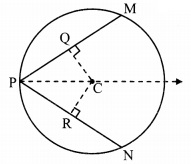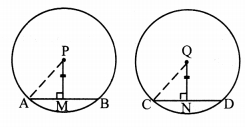# Maharashtra Board 9th Class Maths Part 2 Practice Set 6.2 Solutions Chapter 6 Circle

Balbharti Maharashtra State Board Class 9 Maths Solutions covers the Practice Set 6.2 Geometry 9th Class Maths Part 2 Answers Solutions Chapter 6 Circle.

## Practice Set 6.2 Geometry 9th Std Maths Part 2 Answers Chapter 6 Circle

Question 1.
Radius of circle is 10 cm. There are two chords of length 16 cm each. What will be the distance of these chords from the centre of the circle ?
Given: In a circle with centre O,
OR and OP are radii and RS and PQ are its congruent chords.
PQ = RS= 16 cm,
OR = OP = 10 cm
seg OU ⊥ chord PQ, P-U-Q
seg OT ⊥ chord RS, R-T-S
To find: Distance of chords from centre of the circle.
Solution:i. PU = $$\frac { 1 }{ 2 }$$(PQ) [Perpendicular drawn from the centre of the circle to the chord bisects the chord.]
∴ PU= $$\frac { 1 }{ 2 }$$ x 16 = 8 cm …(i)

ii. In ∆OUP, ∠OUP = 90°
∴ OP2 = OU2 + PU2 [Pythagoras theorem]
∴ 102 = OU2 + 82 [From (i)]
∴ 100 = OU2 + 64
∴ OU2 = 100 – 64 = 36
∴ OU = √36 [Taking square root on both sides]
∴ OU = 6 cm

iii. Now, OT = OU [Congruent chords of a circle are equidistant from the centre.]
∴ OT = OU = 6cm
∴ The distance of the chords from the centre of the circle is 6 cm.

Question 2.
In a circle with radius 13 cm, two equal chords are at a distance of 5 cm from the centre. Find the lengths of chords.
Given: In a circle with cente O,
OA and OC are the radii and AB and CD are its congruent chords,
OA = OC = 13cm
0E = OF = 5 cm
seg 0E ⊥ chord CD, C-E-D
seg OF ⊥ chord AB. A-F-B
To find: length of the chords
Solution:i. In ∆AFO, ∠AFO = 90°
∴ AO2 = AF2 + FO2 [Pythagoras theorem]
∴ 132 = AF2 + 52
∴ 169 = AF2 + 25
∴ AF2 = 169-25
∴ AF2 = 144
∴ AF = $$\sqrt { 144 }$$ [Taking square root on both sides]
∴ AF = 12 cm …..(i)

ii. Now AF = $$\frac { 1 }{ 2 }$$AB [Perpendicular drawn from the centre of the circle to the chord bisects the chord.]
∴ 12 = $$\frac { 1 }{ 2 }$$ (AB) [From (i)]
∴ AB = 12 x 2 = 24 cm
∴ CD = AB = 24 cm [chord AB ≅ chord CD]
∴ The lengths of the two chords are 24 cm each.

Question 3.
Seg PM and seg PN are congruent chords of a circle with centre C. Show that the ray PC is the bisector of ∠NPM.
Given: Point C is the centre of the circle.
chord PM ≅ chord PN
To prove: Ray PC is the bisector of ∠NPM.
Construction: Draw seg CR ⊥ chord PN, P-R-N
seg CQ ⊥ chord PM, P-Q-M
Proof:chord PM chord PN [Given]
seg CR ⊥ chord PN
seg CQ ⊥ chord PM [Construction]
∴ segCR ≅ segCQ ….(i) [Congruent chords are equidistant from the centre]
In ∆PRC and ∆PQC,
∠PRC ≅ ∠PQC [Each is of 90°]
segCR ≅ segCQ [From (i)]
seg PC ≅ seg PC [Common side]
∴ ∆PRC ≅ ∆PQC [Hypotenuse side test]
∴ ∠RPC ≅ ∠QPC [c. a. c. t.]
∴ ∠NPC ≅ ∠MPC [N- R-P, M-Q-P]
∴ Ray PC is the bisector of ∠NPM.

Maharashtra Board Class 9 Maths Chapter 6 Circle Practice Set 6.2 Intext Questions and Activities

Question 1.
Prove the following two theorems for two congruent circles. (Textbook pg. no. 81)
i. Congruent chords in congruent circles are equidistant from their respective centres.
ii. Chords of congruent circles which are equidistant from their respective centres are congruent.
Write ‘Given’. ‘To prove’ and the proofs of these theorems.
Solution:
(i) Congruent chords in congruent circles are equidistant from their respective centres.
Given: Point P and point Q are the centres of congruent circles.
chord AB ≅ chord CD
seg PM ⊥ chord AB, A-M-B
seg QN ⊥ chord CD, C-N-D
To prove: PM = QNConstruction: Draw seg PA and seg QC.
Proof:
seg PM ⊥ chord AB, seg QN ⊥ chord CD [Given]
∴ AM = $$\frac { 1 }{ 2 }$$(AB) ………(i) [Perpendicular drawn from the centre of the circle to the
∴ CN = $$\frac { 1 }{ 2 }$$(CD) ……..(ii) chord bisects the chord.]
But, AB = CD ………(iii) [Given]
∴ AM = CN [From (i), (ii) and (iii)]
i.e., segAM ≅ segCN ….(iv) [Segments of equal lengths]
In ∆PMA and ∆QNC,
∠PMA ≅ ∠QNC [Each is of 90°]
hypotenuse PA ≅ hypotenuse QC [Radii of congruent circles]
seg AM ≅ seg CN [From (iv)]
∴ ∆PMA ≅ ∆QNC [Hypotenuse side test]
∴ segPM ≅ segQN [c. s. c. t.]
∴ PM ≅ QN [Length of congruent segments]

(ii) Chords of congruent circles which are equidistant from their respective centres are congruent.Given: Point P and point Q are the centres of congruent circles.
seg PM ⊥ chord AB, A-M-B
seg QN ⊥ chord CD, C-N-D
PM = QN
To prove: chord AB ≅ chord CD
Construction: Draw seg PA and seg QC.
Proof:
In ∆PMA and ∆QNC,
∴ ∠PMA ≅ ∠QNC [Each is of 90°]
seg PM ≅ seg QN [Given]
hypotenuse PA ≅ hypotenuse QC [Radii of the congruent circles]
∴ ∆PMA ≅ ∆QNC [Hypotenuse side test]
∴ seg AM ≅ seg CN [c. s. c. t.]
∴ AM = CN ….(i) [Length of congruent segments]
Now, seg PM ⊥ chord AB, and seg QN ⊥ chord CD
∴ AM = $$\frac { 1 }{ 2 }$$(AB) …(ii)
∴ CN = $$\frac { 1 }{ 2 }$$ (CD) ..(iii) [Perpendicular drawn from the centre of the circle to the chord bisects the chord.]
∴ AB = CD [From (i), (ii) and (ii)]
∴ chord AB ≅ chord CD [Segments of equal lengths]

Scroll to Top